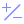#Exponent (PQL)

Returns the number 'e' or Euler's number, raised to the power of the provided value.

• Returned Output: Numeric
• Library: PQL \ Common \ Math
• Version: 2018.00.000
• Compatibility:
• Can be combined with and other PQL function throughout the application.
• It CANNOT be used with MDX or VBA functions. But it can be used on MDX-based content in other parts of the application.

#### Syntax

Exponent( <Numeric> )

* Click on the function's arguments above for more details on the input values.

• The numeric value cane be any type of number: integer, float etc.
##### Different Function types
• This function is like the Excel EXP function.
• Also see the natural log and e functions.

#### Examples

This example returns 2.71828 which is e1

Exponent(1)

This example returns 0.3011942 which e-1.2

Exponent(-1.2)